# Development of New Admittance Matrix for Newton-Raphson Power Flow in Distribution Networks

Development of New Admittance Matrix for Newton-Raphson Power Flow in Distribution Networks

Khaled AlzaareerQusay Salem Claude Ziad El-Bayeh Salman Harasis Al-Motasem I. Aldaoudeyeh Ahmad M.A. Malkawi Ali Q. Al-Shetwi

Electrical Engineering Department, Faculty of Engineering, Philadelphia University, Amman 19392, Jordan

Department of Electrical Engineering, Princess Sumaya University for Technology, Amman 11941, Jordan

Canada Excellence Research Chairs Team, Concordia University, Montreal, Quebec H3G 2J1, Canada

Electrical Power and Mechatronics Engineering Department, Tafila Technical University, Tafila 66110, Jordan

Department of Mechatronics Engineering, The University of Jordan, Amman 11942, Jordan

Department of Electrical Engineering, Fahd bin Sultan University, Tabuk 17454, Saudi Arabia

Corresponding Author Email:
Page:
168-177
|
DOI:
https://doi.org/10.18280/mmep.090121
13 September 2021
|
Accepted:
10 January 2022
|
Published:
28 February 2022
| Citation

OPEN ACCESS

Abstract:

Network modelling is a critical step in the analysis of distribution networks. It is used to relate the input currents and voltages with the output currents and voltages. This work aims to construct a new admittance matrix for distribution networks. The new admittance matrix is derived based on ABCD matrix, which presents the exact model of different types of power distribution networks considering the unbalance/balanced and single/three phase characteristics. The model takes into account shunt admittances to reflect the accurate performances of the components in the distribution networks, especially in the presence of distributed generation units. The importance of considering shunt admittance is due to the presence of capacitive charging current which can affect the node voltages. Application of the new admittance matrix of distribution networks in Newton-Raphson power flow analysis is performed. The standard IEEE 37 bus system is used to test the validity of the proposed approach. MATLAB environment is used to confirm the results. Simulation results show the accuracy of new network admittance matrix in power flow analysis.

Keywords:

power flow, unbalanced system, three-phase networks, distribution networks, admittance matrix, component models

1. Introduction

Electric power networks are one of the most complex systems . Traditionally, power is produced by large power generation units and is transferred to users through transmission lines in a one-way direction. The distribution power systems have been considered as passive networks since the power flow is unidirectional (from the substation to the users). They generally rely on the transmission network for system control. However, the future electrical networks are hosting many loads, distributed generations and ancillary service devices. Such utilization makes distribution systems active due to the power generation inside their networks. Indeed, active networks can supply the excessive power to the high-voltage side. This creates bidirectional power flow in the system. Thus, the network complexity and size are significantly increased. This means that electrical networks are quickly changing and becoming harder to analysis and control .

Network modelling is a crucial stage in analysis of distribution networks. This is due to fact that network modelling can reflect the performance of network components in power system operation . It is used to relate the input currents and voltages with the output currents and voltages . The importance of selecting an appropriate and exact model for a particular power network can be understood in different issues such as power flow analysis, sensitivity analysis, and short circuit analysis. In power system literature, several methods were developed for power flow calculation [5-41], sensitivity analysis [42, 43], and short circuit analysis [44, 45] on distribution networks.

In this work, the authors focus on the issue of power flow computation. Indeed, power flow is an important analysis for future distribution systems. Many network issues like energy management, optimization, state estimation, and network reconfiguration depend on power flow results. Such issues are the heart of system operation, control and planning. Power flow calculation determines the steady state behaviour of the network by computing the voltages magnitudes and phase angles of power networks.

In this regard, this work aims to formulate a new system admittance matrix for distribution networks considering the exact modelling of system components. It deals with the representation of distribution network components under normal operating conditions. The representation can be done by an equivalent model with appropriate fundamental circuit parameters. The component modelling is presented through ABCD matrix, which could simplify the analysis procedure of power network. The new admittance matrix is then utilized in Newton-Raphson based power flow.

The main contribution of this work is to include the exact models of network components in formulating the system admittance matrix. Compared with well-known methods, this method includes the shunt admittances in the analysis.

The rest of this work is organized as follows. Section 2 present the component modelling of different types of distribution power networks. Section 3 presents the new system admittance matrix. Section 4 shows simulation results. The conclusions and future works are stated in Section 5.

2. Modeling of Network Components

This section deals with the representation of distribution network components under normal operating conditions. The representation can be done by an equivalent model with appropriate fundamental circuit parameters. A general form of representing a segment (component) is shown in Figure 1. The segment is between two nodes “n” and “m”. The current passes from node “n” to node “m”.

## 1.png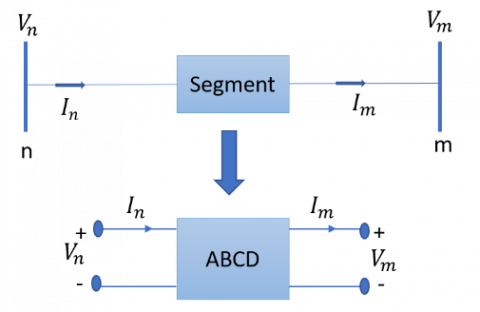Figure 1. Two-port representation of a network segment

By applying Kirchhoff’s voltage and current laws (KVL and KCL), we can obtain the following general equations:

$V_{n}=A V_{m}+B I_{m}$          (1)

$I_{n}=C V_{m}+D I_{m}$             (2)

In matrix form:

$\left[\begin{array}{l}V_{n} \\ I_{n}\end{array}\right]=\left[\begin{array}{ll}A & B \\ C & D\end{array}\right]\left[\begin{array}{l}V_{m} \\ I_{m}\end{array}\right]$        (3)

where, n: sending end of the segment; m: receiving end of the segment; Vn: input voltage; Vm: output voltage; In: input current; Im: output current; ABCD: segment parameters.

Eqns. (1) and (2) represent the relation between the input (voltage & current) and the output (voltage & current) of a segment. The equations can be written in terms of the circuit coefficients (i.e. ABCD constants). Thus, the segment can be represented by a two-port system with ABCD model. According to the type (or characteristics) of distribution networks, network modeling can be classified as presented in the next subsections.

2.1 Single-phase networks (Approximate model)

The representation of single-phase distribution networks can be done by an equivalent model on a per phase basis. The terminal voltage is represented as line to neutral and the current is for one phase.

2.1.1 Distribution line modeling

The distribution overhead and underground line segments in a single-phase distribution network can be approximately modeled by series impedances as shown in Figure 2. This approximation is due to fact that shunt admittances of line segments can be ignored in some cases.

## 2.png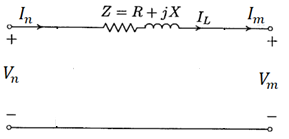Figure 2. Approximate model of a single-phase line

where, Z is the total impedance of the line. R and X represent the total resistance and reactance of the line. The total line impedance can be represented as (r+jwL)l=R+jX. The values; r and L are the per phase resistance and inductance per unit length, respectively. l is the line length. Vn and In are the phase voltage and current at the sending end of the line, and Vm and Im are the phase voltage and current at the receiving end of the line. IL represents the line current.

Set of equations can be developed to model the line segment. For the line segment of Figure 2, the equations that relate the input (node n) voltage and current to the output (node m) voltage and current are developed as follows:

By applying KVL, the phase voltage at the sending end is:

$V_{n}=V_{m}+Z I_{L}$        (4)

By applying KCL, the phase current at the sending end is:

$I_{n}=I_{m}$           (5)

By comparing (4) and (5) with the general model Eqns. (1) and (2), we obtain that A=D=1; C=0; B=Z. In matrix form, we obtain:

$\left[\begin{array}{c}V_{n} \\ I_{n}\end{array}\right]=\left[\begin{array}{ll}1 & Z \\ 0 & 1\end{array}\right]\left[\begin{array}{l}V_{m} \\ I_{m}\end{array}\right]$        (6)

2.1.2 Distribution transformer modeling

The equivalent circuit for a two-winding transformer in a single-phase system can be approximately represented as shown in Figure 3. This approximation is due to fact that shunt admittances of transformers can be ignored in some cases. The equivalent circuit of Figure 3 can be modified by referring the primary impedance (Z1) to the secondary side as shown in Figure 4. It is clear that the equivalent circuit of single-phase transformer consists of an ideal transformer together with elements which represent its real performance.

## 3.pngFigure 3. Approximate model of a single-phase transformer

## 4.pngFigure 4. Modified approximate model of a single-phase transformer

The total impedance Z of the transformer is given by:

$Z=n_{t}^{2} Z_{1}+Z_{2}$

where, nt represent the turn ratio of the transformer and can be calculated as:

$n_{t}=\frac{N_{2}}{N_{1}}$

where, Z1: impedance of the primary winding; Z2: impedance of the secondary winding; R1: resistance of the primary winding; R2: resistance of the secondary winding; X1: reactance of the primary winding; X2: reactance of the secondary winding; N1: number of turns of the primary winding; N2: number of turns of the secondary winding.

Referring to Figure 4, the equation for the ideal transformer becomes:

$E_{2}=n_{t} E_{1}$

Applying KVL in the secondary circuit, we obtain

$E_{2}=V_{m}+Z I_{m}$

The input voltage of the transformer is:

$V_{n}=E_{1}=\frac{1}{n_{t}} E_{2}$

Thus, $V_{n}=\frac{1}{n_{t}} V_{m}+\frac{Z}{n_{t}} I_{m}$            (7)

The input current to the transformer is:

$I_{n}=n_{t} I_{m}$          (8)

By comparing (7) and (8) with the general model equations: we obtain that:

$\left[\begin{array}{l}V_{n} \\ I_{n}\end{array}\right]=\left[\begin{array}{cc}\frac{1}{n_{t}} & \frac{Z}{n_{t}} \\ 0 & n_{t}\end{array}\right]\left[\begin{array}{l}V_{m} \\ I_{m}\end{array}\right]$              (9)

2.2 Single-phase networks (Exact model)

The exact model of single-phase networks can be obtained by including the shunt admittances of line segments and transformers as shown in Figure 5 and Figure 6, respectively. The only difference between the exact and the approximate models is the parameters of ABCD matrix.

## 5.pngFigure 5. Exact model of a single-phase line

where, Y is the total shunt admittance of the line and can be represented as:

$Y=(j w c) l$

where, c is the per phase capacitance per unit length, respectively.

## 6.png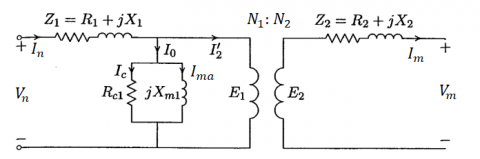Figure 6. Exact model of a single-phase transformer

where, Rc1 and Xm1 are the core resistance and magnetizing reactance, respectively. The transformer shunt admittance Y can be obtained as Rc1//Xm1.

By repeating the same procedure presented in section 2.1.1, we obtain that the matrix ABCD of single-phase lines is changed as:

$\left[\begin{array}{c}V_{n} \\ I_{n}\end{array}\right]=\left[\begin{array}{cc}1+\frac{Z Y}{2} & Z \\ Y\left(1+\frac{Z Y}{4}\right) & 1+\frac{Z Y}{2}\end{array}\right]\left[\begin{array}{l}V_{m} \\ I_{m}\end{array}\right]$              (10)

Similarly, by repeating the same procedure presented in section 2.1.2, we also obtain that the matrix ABCD of single-phase transformers is changed as:

$\left[\begin{array}{c}V_{n} \\ I_{n}\end{array}\right]=\left[\begin{array}{cc}\frac{1}{n_{t}} & \frac{Z}{n_{t}} \\ \frac{Y}{n_{t}} & \frac{Y Z}{n_{t}}+n_{t}\end{array}\right]\left[\begin{array}{c}V_{m} \\ I_{m}\end{array}\right]$            (11)

2.3 Balanced three-phase networks

The representation of balanced three-phase distribution networks can be done by an equivalent model on a “per-phase” basis, similar to models presented in section 2.1. The parameters (Z and Y) are represented per phase. The terminal voltage is presented from line to neutral and the current is for one phase. Thus, the relations expressed in (6), (9) are used for approximate modelling of balanced three phase networks while the expressions illustrated in (10) and (11) are used for exact modelling of balanced three phase networks.

2.4 Unbalanced three-phase networks (Exact model)

For unbalanced systems, it is necessary to involve the actual phasing and the correct spacing between segments (or windings). The impedances and admittances parameters are represented per phase.

2.4.1 Distribution line modelling

The distribution overhead and underground line segments in an unbalanced three-phase distribution network can be represented as shown in Figure 7.

## 7.png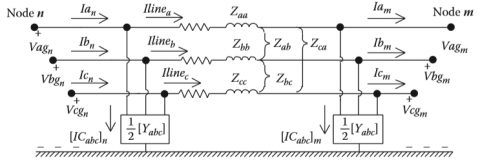Figure 7. Unbalanced three- phase distribution line model

where, Zaa, Zbb, and Zcc are self-impedances for the line a, b and c respectively. Zab, Zbc, and Zca are mutual impedances between the lines. Thus, the impedance matrix Zabc and shunt admittance matrix Yabc for the three phases can be obtained as:

$Z_{a b c}=\left[\begin{array}{lll}Z_{a a} & Z_{a b} & Z_{a c} \\ Z_{b a} & Z_{b b} & Z_{b c} \\ Z_{c a} & Z_{c b} & Z_{c c}\end{array}\right], Y_{a b c}=\left[\begin{array}{lll}Y_{a a} & Y_{a b} & Y_{a c} \\ Y_{b a} & Y_{b b} & Y_{b c} \\ Y_{c a} & Y_{c b} & Y_{c c}\end{array}\right]$

By applying KCL and KVL concepts, we can obtain the ABCD matrix of three-phase lines is as presented in (12)-(15) conclude that:

$\left[\begin{array}{l}V_{n}^{a b c} \\ I_{n}^{a b c}\end{array}\right]=\left[\begin{array}{ll}A & B \\ C & D\end{array}\right]\left[\begin{array}{l}V_{m}^{a b c} \\ I_{m}^{a b c}\end{array}\right]$      (12)

where, $V_{n}^{a b c}=\left[\begin{array}{c}V_{n}^{a g} \\ V_{n}^{b g} \\ V_{n}^{c g}\end{array}\right], V_{m}^{a b c}=\left[\begin{array}{c}V_{m}^{a g} \\ V_{m}^{b g} \\ V_{m}^{c g}\end{array}\right], I_{n}^{a b c}=\left[\begin{array}{c}I_{n}^{a} \\ I_{n}^{b} \\ I_{n}^{c}\end{array}\right]$, $I_{m}^{a b c}=\left[\begin{array}{c}I_{m}^{a} \\ I_{m}^{b} \\ I_{m}^{c}\end{array}\right]$.

From the analysis of KVL and KCL concepts ABCD parameters can be found as:

$A=D=U+\frac{1}{2} Z_{a b c} Y_{a b c}$             (13)

$B=Z_{a b c}$              (14)

$C=Y_{a b c}+\frac{1}{4} Y_{a b c} Z_{a b c} Y_{a b c}$              (15)

where, U is a diagonal matrix and their elements equal to 1.

2.4.2 Distribution transformer modeling

The distribution transformers can be divided into several types based on their winding’s connection. The transformer modelling depends on the winding connection. A general three-phase transformer is shown in Figure 8.

## 8.pngFigure 8. General three-phase transformer bank

By applying KVL and KCL on the transformer terminals, we conclude that:

$\left[\begin{array}{l}V_{n}^{a b c} \\ I_{n}^{a b c}\end{array}\right]=\left[\begin{array}{ll}A & B \\ C & D\end{array}\right]\left[\begin{array}{l}V_{m}^{a b c} \\ I_{m}^{a b c}\end{array}\right]$             (16)

where,

$V_{n}^{a b c}=\left[\begin{array}{l}V_{n}^{A N} \\ V_{n}^{B N} \\ V_{n}^{C N}\end{array}\right], V_{m}^{a b c}=\left[\begin{array}{c}V_{m}^{a n} \\ V_{m}^{b n} \\ V_{m}^{c n}\end{array}\right], I_{n}^{a b c}=\left[\begin{array}{c}I_{n}^{A} \\ I_{n}^{B} \\ I_{n}^{C}\end{array}\right], I_{m}^{a b c}=\left[\begin{array}{c}I_{m}^{a} \\ I_{m}^{b} \\ I_{m}^{c}\end{array}\right]$.

The ABCD parameters of different types of distribution transformer are summarized in Table 1.

where,

$Z_{t a b c}=\left[\begin{array}{ccc}Z_{t a} & 0 & 0 \\ 0 & Z_{t b} & 0 \\ 0 & 0 & Z_{t c}\end{array}\right]$     (17)

$W V^{-1}=\frac{1}{3}\left[\begin{array}{ccc}4 & -2 & 1 \\ 1 & 4 & -2 \\ -2 & 1 & 4\end{array}\right]$      (18)

$W I^{-1}=\frac{1}{3}\left[\begin{array}{lll}2 & 0 & 1 \\ 1 & 2 & 0 \\ 0 & 1 & 2\end{array}\right]$            (19)

The terms VLL and VLNdenote the line- line voltage and line-neutral voltages, respectively. The subscripts p and s represent the primary and secondary winding, respectively. nt is the effective turn ratio. The impedances Zta, Ztb, and Ztc are impedances for Y connected windings. The impedances Ztab, Ztbc, and Ztcaare impedances for Δ connected windings.

Table 1. ABCD parameters of different types of distribution transformer

 Transformer connection ABCD parameters Node n Node m nt A B-1 C D Y-G Y-G $\frac{V_{L N, p}}{V_{L N, s}}$ $n_{t} U$ $\frac{1}{n_{t}}\left[\begin{array}{ccc}Z_{t a} & 0 & 0 \\ 0 & Z_{t b} & 0 \\ 0 & 0 & Z_{t c}\end{array}\right]^{-1}$ 0 $\frac{1}{n_{t}} U$ Y-G Δ $\frac{V_{L N, p}}{V_{L L, s}}$ $n_{t} W V^{-1}$ $\frac{1}{n_{t}}\left[\begin{array}{ccc}\frac{1}{Z_{t a b}} & 0 & \frac{-1}{Z_{t c a}} \\ \frac{-1}{Z_{t a b}} & \frac{1}{Z_{t b c}} & 0 \\ 0 & \frac{-1}{Z_{t b c}} & \frac{1}{Z_{t c a}}\end{array}\right]$ 0 $\frac{1}{n_{t}} W I^{-1}$ Y Δ $\frac{V_{L N, p}}{V_{L L, s}}$ $n_{t} W V^{-1}$ $\frac{1}{n_{t}}\left[\begin{array}{ccc}\frac{1}{Z_{t a b}} & 0 & \frac{-1}{Z_{t c a}} \\ \frac{-1}{Z_{t a b}} & \frac{1}{Z_{t b c}} & 0 \\ 0 & \frac{-1}{Z_{t b c}} & \frac{1}{Z_{t c a}}\end{array}\right]$ 0 $\frac{1}{3 n_{t}}\left[\begin{array}{ccc}2 & 0 & 1 \\ 2 & 3 & 1 \\ -1 & 0 & 1\end{array}\right]$ Δ Y-G $\frac{V_{L L, p}}{V_{L N, s}}$ $\frac{-n_{t}}{3}\left[\begin{array}{lll}0 & 2 & 1 \\ 1 & 0 & 2 \\ 2 & 1 & 0\end{array}\right]$ $\frac{-1}{3 n_{t}}\left[\begin{array}{ccc}\frac{-2}{Z_{t a}} & \frac{1}{Z_{t a}} & \frac{4}{Z_{t a}} \\ \frac{4}{Z_{t b}} & \frac{-2}{Z_{t b}} & \frac{1}{Z_{t b}} \\ \frac{1}{Z_{t c}} & \frac{4}{Z_{t c}} & \frac{-2}{Z_{t c}}\end{array}\right]$ 0 $\frac{1}{n_{t}}\left[\begin{array}{ccc}1 & -1 & 0 \\ 0 & 1 & -1 \\ -1 & 0 & 1\end{array}\right]$ Δ Δ $\frac{V_{L L, p}}{V_{L L, s}}$ $n_{t} U$ $W V^{-1}$ 0 $\frac{1}{n_{t}} U$ Open Y-G (phase c) Open Δ (phase c) $\frac{V_{L N, p}}{V_{L L, s}}$ $n_{t}\left[\begin{array}{ccc}1 & -1 & 0 \\ 0 & 1 & -1 \\ -1 & 0 & 1\end{array}\right]$ $\frac{-1}{3 n_{t}}\left[\begin{array}{ccc}\frac{1}{Z_{t a}} & 0 & 0 \\ \frac{-1}{Z_{t a}} & \frac{1}{Z_{t b}} & 0 \\ 0 & \frac{-1}{Z_{t b}} & 0\end{array}\right]$ $\frac{1}{n_{t}}\left[\begin{array}{ccc}1 & 0 & 0 \\ 0 & 0 & -1 \\ 0 & 0 & 0\end{array}\right]$
3. New Network Admittance Matrix of Distribution Networks

Figure 9 presents a one-line diagram of a balanced three-phase distribution system. The network consists of four buses. The voltage magnitude Vn, phase angle δn, active and reactive power Pn, Qn are the common quantities linked with every bus.

## 9.png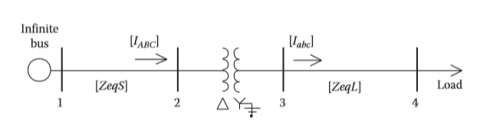Figure 9. One line diagram of a balanced three phase distribution feeder

By applying KCL at each node and considering the general formula illustrated in (2), we obtain:

At node 1 (Where 0 denotes the ground):

$I_{1}=\left(C_{10} V_{1}\right)+\left(C_{12} V_{2}+D_{12} I_{12}\right)$          (20)

At node 2:

$0=\left(C_{21} V_{1}+D_{21} I_{21}\right)+\left(C_{23} V_{3}+D_{23} I_{23}\right)$      (21)

At node 3:

$0=\left(C_{32} V_{2}+D_{32} I_{32}\right)+\left(C_{34} V_{4}+D_{34} I_{34}\right)$        (22)

At node 4:

$-I_{4}=\left(C_{43} V_{3}+D_{43} I_{43}\right)$         (23)

where, the subscripts “n” & “m” of the terms (Cnm, Dnm, and Inm) denote the branch n-m. the subscript “0” denotes the ground. Since the buses 2 and 3 are tie buses, their current injections are zero. Bus 4 is a load bus and therefore it was assigned with a negative value.

The currents Inm can be obtained from the general equation illustrated in (2) as:

$I_{n m}=\frac{V_{n}-A_{n m} V_{m}}{B_{n m}}$        (24)

By substituting (24) into (20), (21), (22) and (23), we obtain

$I_{1}=\left(C_{10}+D_{12} B_{12}^{-1}\right) V_{1}+\left(C_{12}-D_{12} B_{12}^{-1} A_{12}\right) V_{2}$           (25)

\begin{aligned} 0=\left(C_{21}-D_{21} B_{21}^{-1} A_{21}\right) V_{1} & \\ &+\left(D_{21} B_{21}^{-1}+D_{23} B_{23}^{-1}\right) V_{2} \\ &+\left(C_{23}-D_{23} B_{23}^{-1} A_{23}\right) V_{3} \end{aligned}         (26)

\begin{aligned} 0=\left(C_{32}-D_{32} B_{32}^{-1} A_{32}\right) V_{2} & \\+&\left(D_{32} B_{32}^{-1}+D_{34} B_{34}^{-1}\right) V_{3} \\+&\left(C_{34}-D_{34} B_{34}^{-1} A_{34}\right) V_{4} \end{aligned}     (27)

$-I_{4}=\left(C_{43}-D_{43} B_{43}^{-1} A_{43}\right) V_{3}+D_{43} B_{43}^{-1} V_{4}$            (28)

or

$I_{1}=Y_{11} V_{1}+Y_{13} V_{2}+0 V_{3}+0 V_{4}$        (29)

$0=Y_{21} V_{1}+Y_{22} V_{2}+Y_{23} V_{3}+0 V_{4}$      (30)

$0=0 V_{1}+Y_{32} V_{2}+Y_{33} V_{3}+Y_{34} V_{4}$      (31)

$-I_{4}=0 V_{1}+0 V_{2}+Y_{43} V_{3}+Y_{44} V_{4}$         (32)

By comparing (29)-(32) with (25)-(28), we obtain:

$Y_{11}=C_{10}+D_{12} B_{12}^{-1}$        (33.a)

$Y_{22}=D_{21} B_{21}^{-1}+D_{23} B_{23}^{-1}$     (33.b)

$Y_{33}=D_{32} B_{32}-1+D_{34} B_{34}-1$        (33.c)

$Y_{44}=D_{43} B_{43}^{-1}$          (33.d)

$Y_{12}=Y_{21}=C_{12}-D_{12} B_{12}^{-1} A_{12}$         (34.a)

$Y_{23}=Y_{32}=C_{23}-D_{23} B_{23}^{-1} A_{23}$       (34.b)

$Y_{34}=Y_{43}=C_{34}-D_{34} B_{34}^{-1} A_{34}$        (34.c)

$Y_{24}=Y_{42}=Y_{13}=Y_{31}=Y_{14}=Y_{41}=0$       (35)

From (33)-(35), we can conclude a general formula for the elements of system impedance matrix. The diagonal elements can be obtained as:

$Y_{n n}=C_{n 0}+\sum_{m=1}^{N} D_{n m} B_{n m}{ }^{-1}$      (36)

The non-diagonal elements can be obtained as:

$Y_{n m}=Y_{m n}=C_{n m}-D_{n m} B_{n m}{ }^{-1} A_{n m}$        (37)

If there is no connection between the any two buses, then:

$Y_{n m}=0$        (38)

Thus, in general form, the nodal current equations can be written as:

## 39.png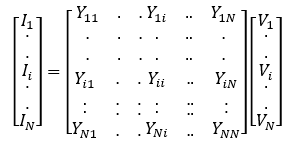(39)

Similarly, for unbalanced three phase distribution networks, The diagonal, non-diagonal elements, and the nodal current equation can be obtained as:

$Y_{n n}^{a b c}=C_{n 0}+\sum_{m=1}^{x} D_{n m} B_{n m}^{-1}$     (40)

$Y_{n m}^{a b c}=Y_{m n}=C_{n m}-D_{n m} B_{n m}^{-1} A_{n m}$        (41)

## 42.png(42)

where, $V_{i}^{a b c}$ and $I_{i}^{a b c}$ are vectors of the three phase voltages and current, respectively, of node i.

The new admittance matrix can be utilized Newton-Raphson power flow for accurate results.

4. Simulation Results

To validate the proposed approach, the new admittance matrix is used for power flow analysis via Newton-Raphson power flow. The new admittance matrix considers the exact model of networks (i.e. series impedance, shunt admittances, ant transformer turn ratio).

## 10.pngFigure 10. The standard IEEE 37 bus network

The standard IEEE 37-bus network shown in Figure 10 was chosen as an unbalanced test network. The power flow and the new admittance matrix algorithms were implemented in MATLAB software. Without loss of generality, only voltage magnitudes are considered for check the accuracy of the ne admittance matrix. Two cases are considered for the analysis as shown in the next subsections.

4.1 At no distributed generation

## 11.pngFigure 11. Voltage profile obtained from power flow results using the new admittance matrix at no distributed generation

Table 2. The percent errors in the voltages at no distributed generation (×10-3)

 Bus Phase a Phase b Phase c 799 0.00 0.00 0.00 701 0.00 0.00 0.00 702 0.14 0.23 0.34 703 0.76 0.51 0.71 727 0.25 0.49 0.77 744 0.22 0.32 0.69 728 0.15 0.14 1.12 729 0.49 0.57 1.02 730 0.11 0.55 0.34 709 0.36 0.65 0.60 708 1.05 1.14 0.66 732 1.20 1.25 1.68 733 0.95 0.56 0.78 734 0.85 0.28 0.85 710 1.80 0.48 1.81 735 1.60 0.48 0.80 736 1.68 1.24 0.91 737 1.19 0.72 0.22 738 1.66 1.08 0.55 711 2.00 0.94 1.08 740 0.46 0.61 0.89 741 1.51 1.69 1.02 731 1.90 2.03 1.93 775 1.28 0.80 0.98 705 0.16 0.12 0.23 712 0.37 0.58 0.43 742 0.24 0.03 0.16 713 0.12 0.04 0.68 704 0.99 0.44 0.27 714 0.97 0.59 0.36 718 0.36 0.74 0.82 720 1.04 0.84 1.00 706 0.67 0.82 0.37 725 0.61 0.12 0.49 707 0.45 0.35 0.86 722 1.66 1.23 1.58 724 1.92 1.80 2.00

By performing the power flow on the test system and by considering the new admittance matrix, the three phase voltages are obtained. Figure 11 shows the three phase voltage profiles. It is clear that the voltage at slack bus equals 1 at the three phases. The results also show that the variation in the voltage from one bus to another bus is directly related to the physical connection between the nodes. The results of Newton-Raphson power flow that consider only the series impedances are also obtained. The percentage errors in the three phase voltages of network buses are presented in Table 2. All the relative errors are in the order of 10-3-10-2, which demonstrate the importance of including the shunt admittances in the analysis for accurate results. Indeed, the exact models of electric power components reflect the charging currents that can be supplied to the network.

4.2 With distributed generation

The effect on the three phase voltages in case of integrating distributed generation into the system is also studied in this work. Two distributed generation units are installed in the system. Their locations are at buses 724 and 722. The capacity of each unit is half its own load. By performing the power flow analysis considering the new admittance matrix, we obtain the three-phase voltage profiles shown in Figure 12. It is clear that the voltages are significantly improved at (and near) the buses 724 and 722. The results are also compared with power flow results without including the shunt admittances. The percent errors in the three phase voltages of network buses in the presence of distributed generation are presented in Table 3. The errors are also in the order of 10-3-10-2. This is due to the fact that including the shunt admittances in the analysis can affect the charging current injected into the network, and hence the bus voltages.

## 12.pngFigure 12. Voltage profile obtained from power flow results using the new admittance matrix in the presence of distributed generation

Table 3. The percent errors in the voltages in the presence of distributed generation (×10-3)

 Bus Phase a Phase b Phase c 799 0.00 0.00 0.00 701 0.00 0.00 0.00 702 0.15 0.25 0.4 703 0.81 0.54 0.8 727 0.27 0.52 0.86 744 0.24 0.34 0.73 728 0.14 0.13 1.18 729 0.55 0.67 1.08 730 0.10 0.58 0.31 709 0.41 0.69 0.55 708 1.17 1.35 0.78 732 1.34 1.48 1.87 733 1.12 0.66 0.87 734 0.95 0.32 0.78 710 2.00 0.54 1.65 735 1.78 0.54 0.95 736 1.77 1.45 1.08 737 1.26 0.84 0.26 738 1.94 1.20 0.62 711 2.23 1.05 1.21 740 0.52 1.01 0.94 741 1.68 1.54 1.08 731 2.21 1.85 2.25 775 1.49 0.94 1.15 705 0.18 0.14 0.26 712 0.42 0.65 0.48 742 0.27 0.04 0.18 713 0.11 0.05 0.62 704 0.9 0.47 0.25 714 0.89 0.63 0.42 718 0.41 0.87 0.92 720 1.16 0.98 1.12 706 0.75 0.92 0.44 725 0.72 0.14 0.45 707 0.53 0.41 1 722 1.94 1.37 1.67 724 2.14 2.01 2.11

By obtaining the average percent errors for each phase voltage, we find that the average errors in case of the presence of distributed generation is higher than the normal case. This also demonstrates the necessity to include such new admittance matrix in the analysis, instead of the conventional matrix. It is worth mentioning that the errors can be higher in case of practical systems or large scale-systems.

5. Conclusion

This work developed a new admittance matrix for distribution network for Newton-Raphson power flow purposes. The new admittance matrix considers the exact models for network components. Newton-Raphson power flow was performed on standard IEEE 37 bus network. The results obtained using the proposed method showed that the voltage profiles among network buses coincide with the physical connection between the nodes. The results also showed that the percent errors (in the voltages obtained using the new admittance matrix and the conventional ones) are in the order of 10-3-10-2. This demonstrates the importance of including such new admittance matrix in the analysis to obtain accurate results. Besides, the average errors in case of presence of distributed generation were greater than in case of passive networks.

References

 Schavemaker, P., Van der Sluis, L. (2017). Electrical Power System Essentials, 2nd Edition. John Wiley & Sons.

 Sereeter, B., Vuik, K., Witteveen, C. (2017). Newton power flow methods for unbalanced three-phase distribution networks. Energies, 10(10): 1658. https://doi.org/10.3390/en10101658

 Kresting, W.H. (2012). Distribution System Modeling and Analysis. Third edition. CRC Press.

 Saadat, H. (2011). Power System Analysis. Third edition. PSA Publishing LLC

 Teng, J.H. (2003). A direct approach for distribution system load flow solutions. IEEE Transactions on Power Delivery, 18(3): 882-887. https://doi.org/10.1109/TPWRD.2003.813818

 Demirok, E., Kjær, S.B., Sera, D., Teodorescu, R. (2012). Three-phase unbalanced load flow tool for distribution networks. In 2nd International Workshop on Integration of Solar Power Systems, Energynautics GmbH, pp. 1-9.

 Maya, K.N., Jasmin, E.A. (2015). A three phase power flow algorithm for distribution network incorporating the impact of distributed generation models. Procedia Technology, 21: 326-331. https://doi.org/10.1016/j.protcy.2015.10.040

 Tang, X.B., Tang, G.Q. (2010). Power flow for distribution network with distributed generation. In 2010 Asia-Pacific Power and Energy Engineering Conference, Chengdu, China, pp. 1-4. https://doi.org/10.1109/APPEEC.2010.5448305

 da Costa, V.M., Martins, N., Pereira, J.L.R. (1999). Developments in the Newton Raphson power flow formulation based on current injections. IEEE Transactions on Power Systems, 14(4): 1320-1326. https://doi.org/10.1109/59.801891

 Murari, K., Padhy, N.P. (2020). Graph-theoretic based approach for the load-flow solution of three-phase distribution network in the presence of distributed generations. IET Generation, Transmission & Distribution, 14(9): 1627-1640.

 Khushalani, S., Solanki, J.M., Schulz, N.N. (2007). Development of three-phase unbalanced power flow using PV and PQ models for distributed generation and study of the impact of DG models. IEEE Transactions on Power Systems, 22(3): 1019-1025. https://doi.org/10.1109/TPWRS.2007.901476

 Thukaram, D.H.M.W., Banda, H.W., Jerome, J. (1999). A robust three phase power flow algorithm for radial distribution systems. Electric Power Systems Research, 50(3): 227-236. https://doi.org/10.1016/S0378-7796(98)00150-3

 Cheng, C.S., Shirmohammadi, D. (1995). A three-phase power flow method for real-time distribution system analysis. IEEE Transactions on Power systems, 10(2): 671-679. https://doi.org/10.1109/59.387902

 Moghaddas-Tafreshi, S.M., Mashhour, E. (2009). Distributed generation modeling for power flow studies and a three-phase unbalanced power flow solution for radial distribution systems considering distributed generation. Electric Power Systems Research, 79(4): 680-686. https://doi.org/10.1016/j.epsr.2008.10.003

 Ranjan, R., Venkatesh, B., Chaturvedi, A., Das, D. (2004). Power flow solution of three-phase unbalanced radial distribution network. Electric Power Components and Systems, 32(4): 421-433. https://doi.org/10.1080/15325000490217452

 Luo, G.X., Semlyen, A. (1990). Efficient load flow for large weakly meshed networks. IEEE Transactions on Power Systems, 5(4): 1309-1316. https://doi.org/10.1109/59.99382

 Haque, M.H. (1996). Efficient load flow method for distribution systems with radial or mesh configuration. IEE Proceedings-Generation, Transmission and Distribution, 143(1): 33-38. https://doi.org/10.1049/ip-gtd:19960045

 Haque, M.H. (2000). A general load flow method for distribution systems. Electric Power Systems Research, 54(1): 47-54. https://doi.org/10.1016/S0378-7796(99)00065-6

 Chiang, H.D. (1991). A decoupled load flow method for distribution power networks: Algorithms, analysis and convergence study. International Journal of Electrical Power & Energy Systems, 13(3): 130-138. https://doi.org/10.1016/0142-0615(91)90001-C

 Chen, T.H., Chen, M.S., Hwang, K.J., Kotas, P., Chebli, E.A. (1991). Distribution system power flow analysis-a rigid approach. IEEE Transactions on Power Delivery, 6(3): 1146-1152. https://doi.org/10.1109/61.85860

 Chen, T.H., Chen, M.S., Inoue, T., Kotas, P., Chebli, E.A. (1991). Three-phase cogenerator and transformer models for distribution system analysis. IEEE Transactions on Power Delivery, 6(4): 1671-1681. https://doi.org/10.1109/61.97706

 Zhang, F., Cheng, C.S. (1997). A modified Newton method for radial distribution system power flow analysis. IEEE Transactions on Power Systems, 12(1): 389-397. https://doi.org/10.1109/59.575728

 Nguyen, H.L. (1997). Newton-Raphson method in complex form. IEEE Power Engineering Review, 17(8): 62-62.

 Alzaareer, K., Saad, M. (2018). Real-time voltage stability monitoring in smart distribution grids. In 2018 International Conference on Renewable Energy and Power Engineering (REPE), pp. 13-17. https://doi.org/10.1109/REPE.2018.8657671

 Garcia, P.A., Pereira, J.L.R., Carneiro, S., Da Costa, V.M., Martins, N. (2000). Three-phase power flow calculations using the current injection method. IEEE Transactions on Power Systems, 15(2): 508-514. https://doi.org/10.1109/59.867133

 Lin, W.M., Teng, J.H. (2000). Three-phase distribution network fast-decoupled power flow solutions. International Journal of Electrical Power & Energy Systems, 22(5): 375-380. https://doi.org/10.1016/S0142-0615(00)00002-8

 Teng, J.H., Chang, C.Y. (2002). A novel and fast three-phase load flow for unbalanced radial distribution systems. IEEE Transactions on Power Systems, 17(4): 1238-1244. https://doi.org/10.1109/TPWRS.2002.805012

 Teng, J.H. (2002). A modified Gauss–Seidel algorithm of three-phase power flow analysis in distribution networks. International Journal of Electrical Power & Energy Systems, 24(2): 97-102. https://doi.org/10.1016/S0142-0615(01)00022-9

 Vieira, J.C.M., Freitas, W., Morelato, A. (2004). Phase-decoupled method for three-phase power-flow analysis of unbalanced distribution systems. IEE Proceedings-Generation, Transmission and Distribution, 151(5): 568-574.

 Chen, T.H., Yang, N.C. (2010). Loop frame of reference based three-phase power flow for unbalanced radial distribution systems. Electric Power Systems Research, 80(7): 799-806. https://doi.org/10.1016/j.epsr.2009.12.006

 Sun, H., Nikovski, D., Ohno, T., Takano, T., Kojima, Y. (2011). A fast and robust load flow method for distribution systems with distributed generations. Energy Procedia, 12: 236-244. https://doi.org/10.1016/j.egypro.2011.10.033

 Lagace, P.J. (2012). Power flow methods for improving convergence. In IECON 2012-38th Annual Conference on IEEE Industrial Electronics Society, Montreal, QC, Canada, pp. 1387-1392. https://doi.org/10.1109/IECON.2012.6388538

 Abdelaziz, M.M.A., Farag, H.E., El-Saadany, E.F., Mohamed, Y.A.R.I. (2012). A novel and generalized three-phase power flow algorithm for islanded microgrids using a newton trust region method. IEEE Transactions on Power Systems, 28(1): 190-201. https://doi.org/10.1109/TPWRS.2012.2195785

 Idema, R., Lahaye, D. (2014). Computational Methods in Power System Analysis. Atlantis Studies in Scientific Computing in Electromagnetics, Atlantis Press: Amsterdam, The Netherlands. https://doi.org/10.2991/978-94-6239-064-5

 Li, C., Chaudhary, S.K., Vasquez, J.C., Guerrero, J.M. (2014). Power flow analysis algorithm for islanded LV microgrids including distributed generator units with droop control and virtual impedance loop. In 2014 IEEE Applied Power Electronics Conference and Exposition-APEC 2014, Fort Worth, TX, USA, pp. 3181-3185. https://doi.org/10.1109/APEC.2014.6803760

 Wang, L., Chen, C., Shen, T. (2014). Improvement of power flow calculation with optimization factor based on current injection method. Discrete Dynamics in Nature and Society, 2014: 437567. https://doi.org/10.1155/2014/437567

 Milano, F. (2015). Analogy and convergence of Levenberg’s and Lyapunov-based methods for power flow analysis. IEEE Transactions on Power Systems, 31(2): 1663-1664. https://doi.org/10.1109/TPWRS.2015.2415455

 Mumtaz, F., Syed, M.H., Al Hosani, M., Zeineldin, H.H. (2015). A novel approach to solve power flow for islanded microgrids using modified Newton Raphson with droop control of DG. IEEE Transactions on Sustainable Energy, 7(2): 493-503. https://doi.org/10.1109/TSTE.2015.2502482

 Pourbagher, R., Derakhshandeh, S.Y. (2018). A powerful method for solving the power flow problem in the ill-conditioned systems. International Journal of Electrical Power & Energy Systems, 94: 88-96. https://doi.org/10.1016/j.ijepes.2017.06.032

 Sereeter, B., Vuik, C., Witteveen, C. (2019). On a comparison of Newton–Raphson solvers for power flow problems. Journal of Computational and Applied Mathematics, 360: 157-169. https://doi.org/10.1016/j.cam.2019.04.007

 Sereeter, B., Vuik, K., Witteveen, C. (2017). Newton power flow methods for unbalanced three-phase distribution networks. Energies, 10(10): 1658. https://doi.org/10.3390/en10101658

 Džafić, I., Jabr, R.A., Halilovic, E., Pal, B.C. (2013). A sensitivity approach to model local voltage controllers in distribution networks. IEEE Transactions on Power Systems, 29(3): 1419-1428. https://doi.org/10.1109/TPWRS.2013.2290813

 Conti, S., Raiti, S., Vagliasindi, G. (2010). Voltage sensitivity analysis in radial MV distribution networks using constant current models. In 2010 IEEE International Symposium on Industrial Electronics, Bari, Italy, pp. 2548-2554. https://doi.org/10.1109/ISIE.2010.5637545

 Youssef, K.H., Abouelenin, F.M. (2016). Analysis of simultaneous unbalanced short circuit and open conductor faults in power systems with untransposed lines and six-phase sections. Alexandria Engineering Journal, 55(1): 369-377. https://doi.org/10.1016/j.aej.2016.01.020

 Kim, I. (2019). Short-circuit analysis models for unbalanced inverter-based distributed generation sources and loads. IEEE Transactions on Power Systems, 34(5): 3515-3526. https://doi.org/10.1109/TPWRS.2019.290355